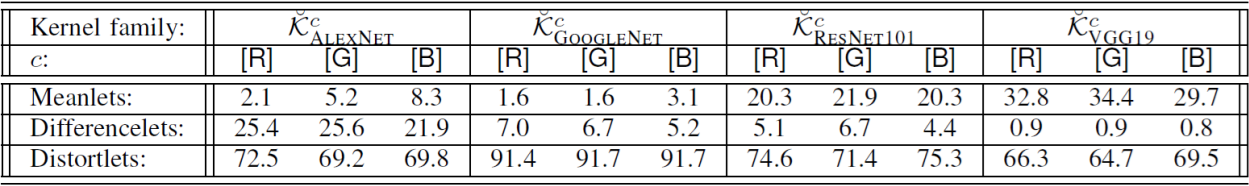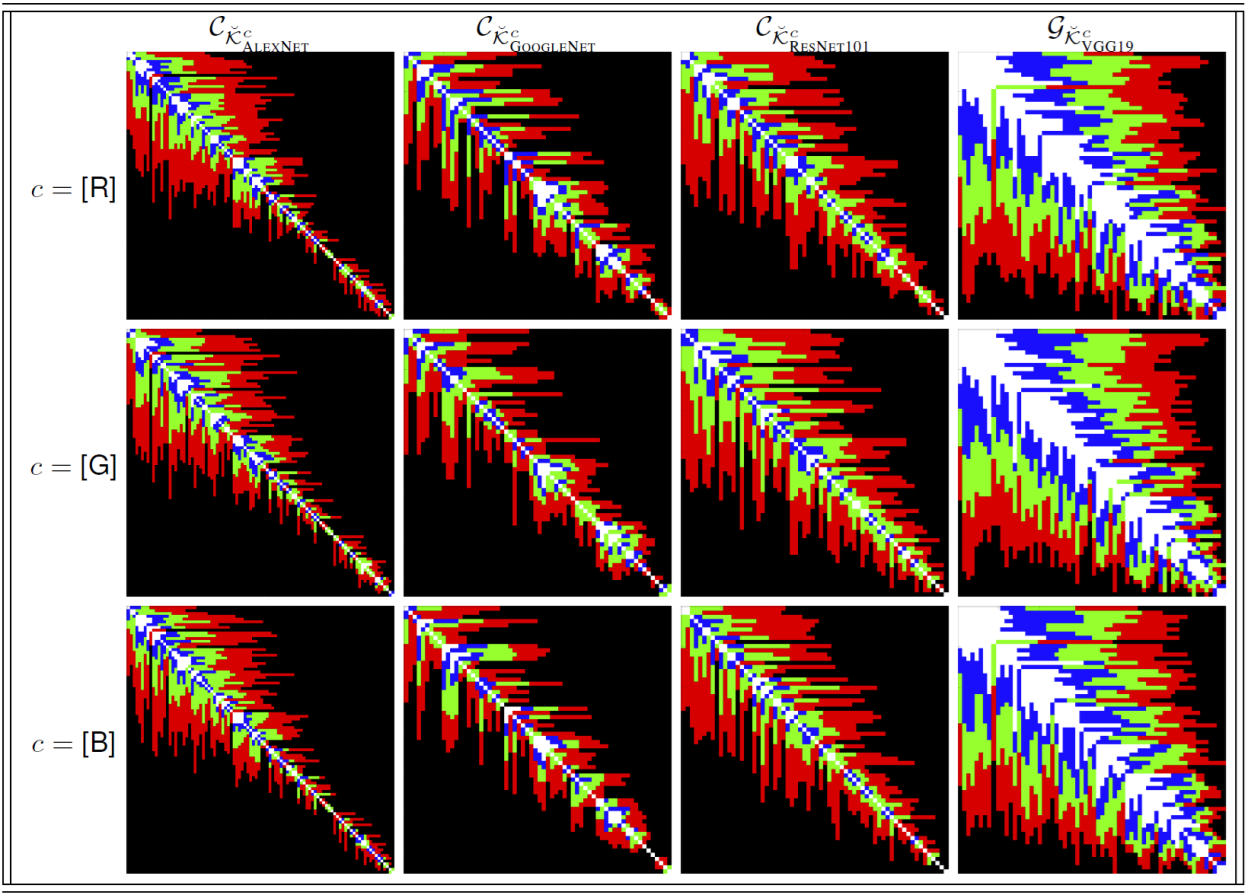Frame theory and Convolutional Neural Networks (CNNs)

CNNs perform penalized harmonic analysis thanks to convolutional (learned) and neural (usually fixed a priori) operators.  Convolutional kernels learned by CNNs can be classified in 3 main categories: Meanlets (penalized mean operators, behaving as weighted means, smoothing kernels or scaling functions), Differencelets (penalized differencing operators sharing similar properties as wavelets and edge detectors) and Distortlets (penalized distortion operators: neither meanlets, nor differencelets), see reference  for details.

 Frames Learned by Prime Convolution Layers in a Deep Learning Framework, IEEE TNNLS, 2020

Rference  provides some harmonic content analysis, frame operator properties and intra-layer correlation structures of convolutional kernels (that are discovered during training of ImageNet) by CNNs such as ALEXNET, GOOGLENET, RESNET101 and VGG19.

Example of a Table showing the distribution (in percentage) of harmonic content for layer #1 convolution kernels in R-G-B.Example of intra-layer inter-kernel correlation structures in R-G-BALEXNET and GOOGLENET show less correlation between layer #1 convolution kernels than RESNET101 and VGG19. This implies more ‘redundancy’ for RESNET101 and VGG19 when passing layer #1.

Conclusion

The distribution of harmonic content, as well as other statistics provided by  can help understanding CNN properties such as generalizability and abstraction capabilities.